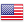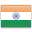Grade "A+" Accredited by NAAC with a CGPA of 3.46
Grade "A+" Accredited by NAAC with a CGPA of 3.46

# Managerial Economics

Course ID
MC 202
Level
Program
BMS
Semester
Second
Credits
6.0
Paper Type
Core Course
Method
Lecture & Tutorial

## Unique Paper Code: 61011203

The purpose of this course is to apply micro economic concepts and techniques in evaluating business decisions taken by firms. The emphasis is on explaining how tools of standard price theory can be employed to formulate a decision problem, evaluate alternative courses of action and finally choose among alternatives.

## Learning Outcomes:

At the end of the course, students should be able to:

• Apply the knowledge of the mechanics of supply and demand to explain working of markets
• Describe how changes in demand and supply affect markets
• Understand the choices made by a rational consumer
• Explain relationships between production and costs
• Define key characteristics and consequences of different forms of markets

## Course Contents

Unit I
Unit II
Unit III
Unit IV
Unit V

Unit I (1 week)

Demand, Supply and Market equilibrium: Individual demand, market demand, individual supply, market supply, market equilibrium; Elasticities of demand and supply; Price elasticity of demand, income elasticity of demand, cross price elasticity of demand, elasticity of supply

References:

Dominick Salvatore (2009). Principles of Microeconomics (5th Edition). Oxford University Press (Chapter 2 and Chapter 5)

Unit II (2 weeks)

Theory of consumer behaviour Cardinal utility theory; ordinal utility theory (indifference curves, budget line, consumer choice, price effect, substitution effect, income effect for normal, inferior and giffen goods); derivation of indifference curves using revealed preference theory.

References:

Dominick Salvatore (2009). Principles of Microeconomics (5th Edition). Oxford University Press (Chapter 3 and Chapter 4)

Unit III (3 weeks)

Producer and optimal production choice Optimizing behaviour in short run (product curves, law of diminishing margin productivity, stages of production); optimizing behaviour in long run (isoquants, isocost line, optimal combination of resources); traditional theory of cost (short run and long run); modern theory of cost (short run and long run); economies of scale and scope.

References:

Dominick Salvatore (2009). Principles of Microeconomics (5th Edition). Oxford University Press (Chapter 7 and Chapter 8)

Unit IV (4 weeks)

Theory of firm and market organization Perfect competition (basic features, short run equilibrium of firm/industry, long run equilibrium of firm/industry, effect of changes in demand, cost and imposition of taxes) ; monopoly (basic features, short run equilibrium, long run equilibrium, effect of changes in demand, cost and imposition of taxes, comparison with perfect competition, welfare cost of monopoly), price discrimination, multiplant monopoly ; monopolistic competition (basic features, demand and cost, short run equilibrium, long run equilibrium, excess capacity) ; oligopoly (Cournot’s model, kinked demand curve model, dominant price leadership model, Game Theory; Oligopolistic behaviour (dominant strategy, Nash equilibrium, business dilemma, pure strategy, mixed strategy, pure strategy equilibrium, mixed strategy equilibrium)

References:

Pindyck, Rubinfeld and Mehta (2009). Micro Economics (7th Edition). Pearson. (Chapter 7, Chapter 9, Chapter 10, Chapter 11 and Chapter 12)

Unit V (2 weeks)

Concept of General Equilibrium Partial vs General Equilibrium; General equilibrium of exchange and production; General equilibrium of exchange and production and Pareto optimality

References:

Dominick Salvatore (2009). Principles of Microeconomics (5th Edition). Oxford University Press (Chapter 16)

#### Text Books

Dominick Salvatore (2009). Principles of Microeconomics (5th Edition). Oxford University Press
Pindyck, Rubinfeld and Mehta (2009). Micro Economics (7th Edition). Pearson.

Lipsey and Chrystal (2008). Economics. (11th Edition). Oxford University Press

#### Teaching Learning Process

Lectures, problems and numerical, team paper, presentations, case studies

#### Assessment Methods

Internal assessment 25 marks
Written theory exam 75 marks

#### Keywords

Equilibrium, rationality, utility maximization, profit maximization, general equilibrium, Pareto optimality

Disclaimer: Details on this page are subject to change as per University of Delhi guidelines. For latest update in this regard please refer to the University of Delhi website here.Englishहिन्दी